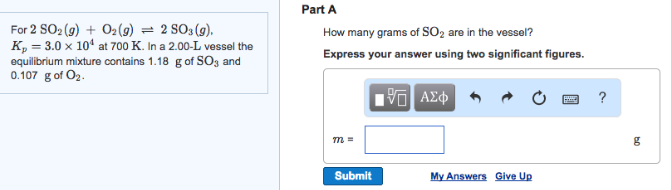# Problem: For 2SO2 (g) + O2 (g) ⇌ 2SO3 (g), Kp = 3.0 x 10 4 at 700 K. In a 2.00-L vessel the equilibrium mixture contains 1.18 g of SO3 and 0.107 g of O2. How many grams of SO2 are in the vessel?Express your answer using two significant figures.

###### FREE Expert Solution
86% (226 ratings)###### Problem Details

For 2SO2 (g) + O2 (g) ⇌ 2SO3 (g), Kp = 3.0 x 10 4 at 700 K. In a 2.00-L vessel the equilibrium mixture contains 1.18 g of SO3 and 0.107 g of O2

How many grams of SO2 are in the vessel?